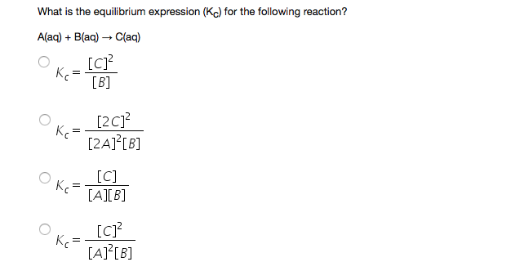Chemistry Practice Problems Equilibrium Expressions Practice Problems Solution: What is the equilibrium expression (Kc) for the fo...

🤓 Based on our data, we think this question is relevant for Professor Reeves's class at Stockton University.

# Solution: What is the equilibrium expression (Kc) for the following reaction? A(aq) + B(aq) → C(aq)

###### Problem

What is the equilibrium expression (Kc) for the following reaction?

A(aq) + B(aq) → C(aq)View Complete Written Solution

Equilibrium Expressions

Equilibrium Expressions

#### Q. Write the equilibrium expression for each of the following dissolution reactions: (a) PbCrO4 (s) ⇌ Pb2+ (aq) + CrO42- (aq)(b) Cs2SO4 (s) ⇌ 2Cs+ (aq) +...

Solved • Tue Jul 17 2018 13:34:41 GMT-0400 (EDT)

Equilibrium Expressions

#### Q. The equilibrium for the reaction for the decomposition of PCl5 to chlorine and PCl3 is 0.042. PCl5(g) ⇌ PCl3(g) + Cl2(g) If the equilibrium concentrat...

Solved • Mon Jul 16 2018 14:44:08 GMT-0400 (EDT)

Equilibrium Expressions

#### Q. What is the numerical value of Kc for the following reaction if the equilibrium mixture contains 0.025 M N2O4 and 0.20 M NO2? N2O4 (g) ⇌ 2NO2 (g) Expr...

Solved • Mon Jul 16 2018 14:40:38 GMT-0400 (EDT)

Equilibrium Expressions

#### Q. The elementary reaction 2H2O(g) ⇌ 2H2(g) + O2(g) proceeds at a certain temperature until the partial pressures of H2O, H2, and O2 reach 0.0550 atm, 0....

Solved • Mon Jul 16 2018 13:09:51 GMT-0400 (EDT)